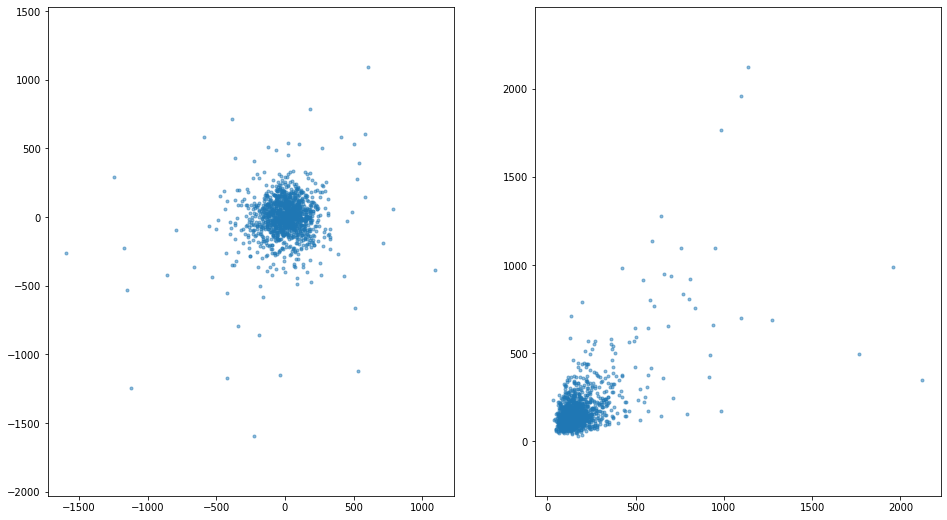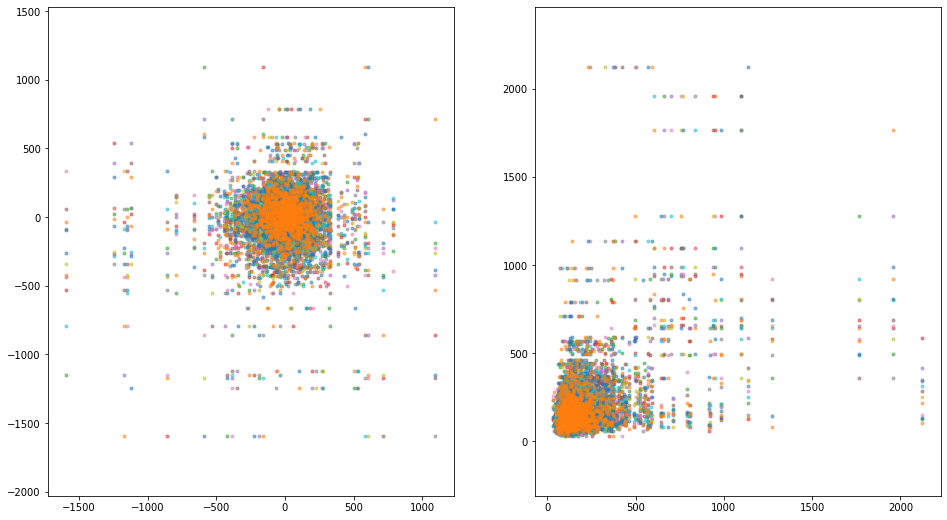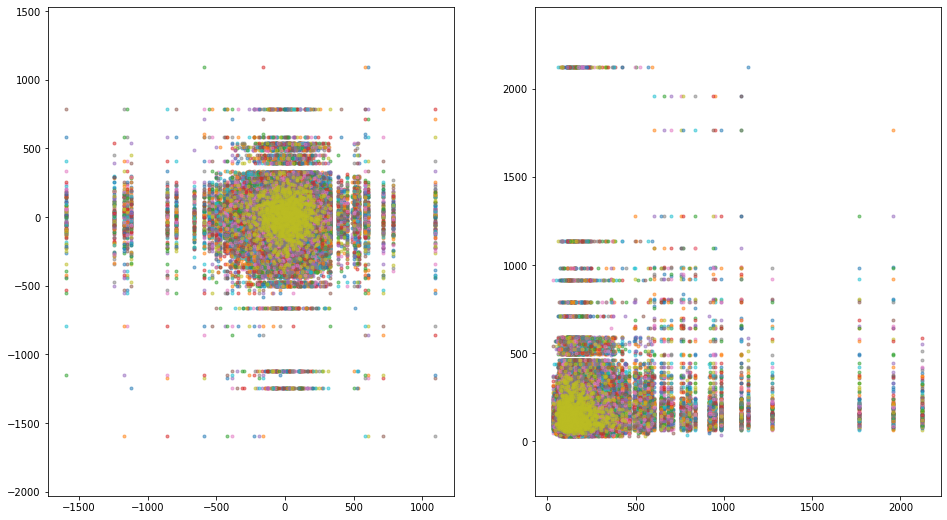# Strange Attractors in Stocks¶

Michal Grochmal mike@grochmal.org

In this notebook we will play with some stock data using elements from chaos theory. For a start let's import a handful of things.

In :
import numpy as np
import matplotlib.pyplot as plt


We will use FTSE250 stock, and we will take as much of it as we can. We will use stooq stock data, the website kindly provides downloads of data for several indexes and several tickers.

In :
df = data.DataReader('^FTM', 'stooq')  # That's FTSE250


Let's see what we got

In :
df

Out:
Open High Low Close Volume
Date
2020-03-30 14784.78 14784.90 14180.67 14624.63 NaN
2020-03-27 15355.39 15355.39 14589.55 14769.80 NaN
2020-03-26 14799.69 15380.71 14545.51 15380.71 NaN
2020-03-25 14212.91 14970.74 14212.91 14819.91 NaN
2020-03-24 13078.01 14172.73 13078.01 14172.73 NaN
... ... ... ... ... ...
2015-04-10 17715.54 17875.11 17715.54 17875.11 245625776.0
2015-04-09 17561.47 17715.54 17561.07 17715.54 257184160.0
2015-04-08 17521.48 17627.43 17521.23 17561.47 269268672.0
2015-04-07 17268.83 17521.48 17268.62 17521.48 265682528.0
2015-04-02 17123.41 17268.83 17123.41 17268.83 225564992.0

1263 rows × 5 columns

OK, we have 5 years of basic stock data. It is pretty basic, it is only the aggregated points of the entire day. That should be enough for our purposes though.

Let's see the difference that a each day trading has performed. We will be working under the (incorrect) assumption that one day's open value is the previous day closing value but that should also be good enough for our purposes.

In :
series = df.Close - df.Open


Another option is to take a differential as the maximum variance that happened during the day. This would be the highest value minus the lowest value during the day.

In :
svar = df.High - df.Low


We will argue that the difference in a day is a differential. In other words, has we take the derivative of the entire stock run over the entire curve and then take a specific day to look at we would get a value pretty similar to close value minus the open value.

Also, since stock values are results of a complex process we will ignore the variables causing the process. What we will argue is that the process generating the stock values is recursive. In simple words, we will argue that anyone trading today is basing his bid and ask decisions by the current prices and recent past prices - the common idea of a "trend". In other words, we are arguing that stock prices are generated by some process that can be modeled by a set of recursive differential equations - a dynamical system. We cannot and will not attempt to discover what the equations are, we will only attempt to argue that such a system of equations exists and is recursive.

For a start let's make a simple graph superposing the current day trading (close value minus open value) on the $x$ axis, against the trading value of the next day, on the $y$ axis.

In :
x1 = series.to_numpy()
x2 = svar.to_numpy()
fig, (ax1, ax2) = plt.subplots(1, 2, figsize=(16, 9))
ax1.plot(x1[:-1], x1[1:], '.', alpha=.5)
ax2.plot(x2[:-1], x2[1:], '.', alpha=.5)
ax1.axis('equal')
ax2.axis('equal');One with experience in statistics will argue that we have a Gaussian blob due to the central limit theorem (CLT). In other words, that there is a center at zero and there are vanishing tails in all directions.

We will argue that that argument is untrue. Had we had a Gaussian (CLT) case in our stock values we would need a continuous Gaussian distribution of randomness, yet this is not the case. The previous graph does not show the structure of the data because we have too few points. If we compare each day against a day 2 days later, then against a day 3 days later, and so on; we will see that there is structure in the data.

In :
x1 = series.to_numpy()
x2 = svar.to_numpy()
fig, (ax1, ax2) = plt.subplots(1, 2, figsize=(16, 9))
ax1.axis('equal')
ax2.axis('equal')

ax1.plot(x1[:-1], x1[1:], '.', alpha=.5)
ax1.plot(x1[:-2], x1[2:], '.', alpha=.5)
ax1.plot(x1[:-3], x1[3:], '.', alpha=.5)
ax1.plot(x1[:-4], x1[4:], '.', alpha=.5)
ax1.plot(x1[:-5], x1[5:], '.', alpha=.5)
ax1.plot(x1[:-6], x1[6:], '.', alpha=.5)
ax1.plot(x1[:-7], x1[7:], '.', alpha=.5)
ax1.plot(x1[:-8], x1[8:], '.', alpha=.5)
ax1.plot(x1[:-9], x1[9:], '.', alpha=.5)
ax1.plot(x1[:-10], x1[10:], '.', alpha=.5)
ax1.plot(x1[:-11], x1[11:], '.', alpha=.5)
ax1.plot(x1[:-12], x1[12:], '.', alpha=.5)

ax2.plot(x2[:-1], x2[1:], '.', alpha=.5)
ax2.plot(x2[:-2], x2[2:], '.', alpha=.5)
ax2.plot(x2[:-3], x2[3:], '.', alpha=.5)
ax2.plot(x2[:-4], x2[4:], '.', alpha=.5)
ax2.plot(x2[:-5], x2[5:], '.', alpha=.5)
ax2.plot(x2[:-6], x2[6:], '.', alpha=.5)
ax2.plot(x2[:-7], x2[7:], '.', alpha=.5)
ax2.plot(x2[:-8], x2[8:], '.', alpha=.5)
ax2.plot(x2[:-9], x2[9:], '.', alpha=.5)
ax2.plot(x2[:-10], x2[10:], '.', alpha=.5)
ax2.plot(x2[:-11], x2[11:], '.', alpha=.5)
ax2.plot(x2[:-12], x2[12:], '.', alpha=.5);Oh! If you never looked at chaos theory we are confident that that was unexpected. There is structure where one expects randomness!

In chaos theory we call this structure a projection of a strange attractor. A strange attractor is the structure of the process evolution in phase space, i.e. given all degrees of freedom the system moves over the strange attractor structure at each time step (here days). We do not know what is dimensionality of the strange attractor, notably because we do not know all the degrees of freedom of the system we are looking at (stock price). Despite the we can see the structure of the projection of the attractor in the two dimensional graph.

If we add more and more points to our graph the structure does not disappear. Instead, the structure becomes clearer. We can argue that the structure is fractal, as we move from the zero the holes in the structure increase in size. The center of the structure does have the same structure, with holes at smaller and smaller scales, but we cannot see it on this graph due to the size of the plot.

In :
x1 = series.to_numpy()
x2 = svar.to_numpy()
fig, (ax1, ax2) = plt.subplots(1, 2, figsize=(16, 9))
ax1.axis('equal')
ax2.axis('equal')
for i in range(1, 120):
ax1.plot(x1[:-i], x1[i:], '.', alpha=.5)
ax2.plot(x2[:-i], x2[i:], '.', alpha=.5)Hey! Hey! You're playing tricks here, this must be special to FTSE250!

Well, yes, this specific structure is specific to FTSE250. Yet, other stocks will present a very similar structure. First because the general stock value process is always recursive. Second because stock value are linked by bids and asks of people (and organizations) trading on several stocks at once.

Let's take the UK100 stock value instead:

In :
df = data.DataReader('^UKX', 'stooq')  # That's UK100
x = (df.Close - df.Open).to_numpy()


And take the same graph, with a lot of points from the start.

In :
fig, (ax1, ax2) = plt.subplots(1, 2, figsize=(16, 9))
ax1.axis('equal')
ax2.axis('equal')
for i in range(1, 120):
ax1.plot(x1[:-i], x1[i:], '.', alpha=.5)
ax2.plot(x2[:-i], x2[i:], '.', alpha=.5)We have a very similar structure. This structure comes from the recursive behavior of stock asks and bids, therefore every stock will have a similar structure given enough data points.

But what is this useful for? For a start, the interesting parts of the projected strange attractor are the holes - the places where there are no points. For example, we can argue that in UK100 we will never see a day closing $400$ or $600$ points away from its opening position. Why is that? The graph does not offer an explanation, although we can speculate. One could say that the market forces (whatever they are) combine as a dynamical system in a way that: whenever a closing value may be $-400$ the score will be attracted to the line at $-510$ or the line at $-290$. That said, we cannot say a thing how these forces act in order to be attracted away from $-400$.

The strange attractor is a product of the recursive nature of the process. One can find such attractor in many places in the real world. For example, a the technique used here to show the attractors was first conceived to visualize the strange attractor for a dripping tap. I hope this may stir your interest in searching for strange attractors in your data, and that you remember the complexity can arise very quickly in recursive systems.